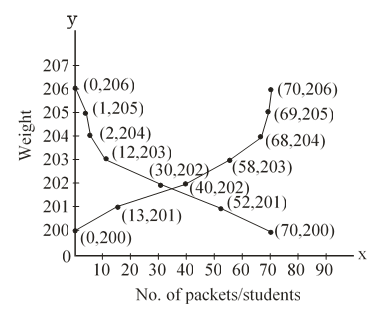#### The weights of tea in 70 packets are shown in the following table :  Weight (in gram) Number of packets 200-201 201-202 202-203 203-204 204-205 205-206 13 27 18 10 1 1  Draw the less than type and more than type ogives for the data and use them to find the median weight.Solution.

 Less than type More than type Weight Number of packets Number of packets Number of students Less than 200 0 More than or equal to 200 70 Less than 201 13 More than or equal to 201 70-13 = 57 Less than 202 40 More than or equal to 202 57-27 =30 Less than 203 58 More than or equal to 203 30-18 =12 Less than 204 68 More than or equal to 204 12-10 = 2 Less than 205 69 More than or equal to 205 2-1 = 1 Less than 206 70 More than or equal to 206 1-1 = 0Hence median = 201.8